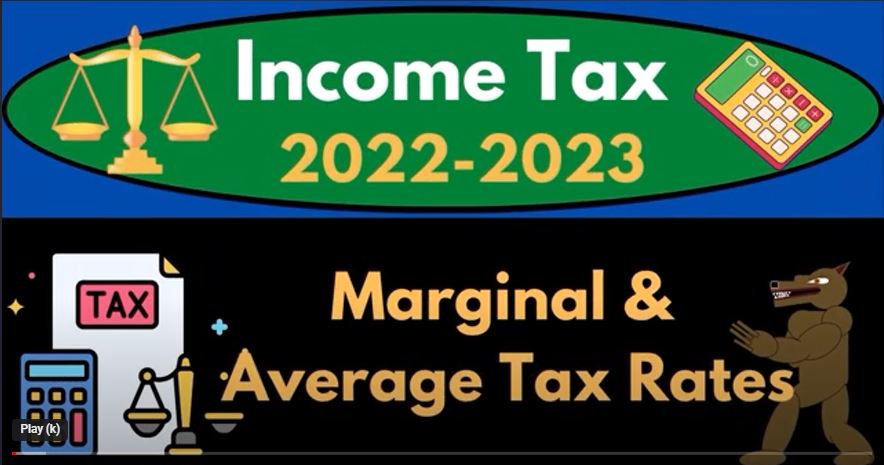## Marginal & Average Tax Rates 1070 Income Tax Preparation 2022 – 2023

Understanding income tax can be complex, especially in a progressive tax system like that in the United States. As income increases, so do the tax rates, resulting in multiple tiers or layers of taxes that make the calculation more complex. This complexity is why it’s important to understand the concepts of marginal and average tax rates, which are key to tax preparation and wealth preservation.

The United States income tax is a progressive tax system, which means that the tax rate increases as the taxable income increases. To give you an idea, here are the 2023 single filer tax brackets:

• 10% on taxable income from \$0 to \$11,000
• 12% on taxable income from \$11,001 to \$44,725
• 22% on taxable income from \$44,726 to \$95,375
• 24% on taxable income from \$95,376 to \$195,725
• 32% on taxable income from \$195,726 to \$305,325
• 35% on taxable income from \$305,326 to \$410,700
• 37% on taxable income over \$410,700

To calculate income tax, we need to determine the taxable income, which is the income after all allowable deductions have been subtracted from gross income. The tax software then applies the appropriate tax rate based on the taxable income amount.

The complexity of multiple tax rates leads to a need for a way to summarize those rates in one number, which is where the concepts of average tax and marginal tax rates come in.

The average tax rate is the total tax paid divided by the taxable income. For example, if a person’s taxable income is \$100,000 and they paid \$20,000 in taxes, their average tax rate would be 20%. This number gives a summary of the person’s overall tax burden.

However, the average tax rate is not the best rate to use for planning into the future because it’s an average of all tax rates applied to different income levels. The marginal tax rate, on the other hand, is the tax rate that applies to the next dollar of taxable income earned. It’s important for planning because it tells you what tax rate you’ll be paying on the additional income you’re expecting to earn.

For example, if a person’s taxable income is \$100,000 and they’re expecting to earn an additional \$10,000, the marginal tax rate will tell them what tax rate they’ll be paying on that \$10,000. If their taxable income is already in the 24% bracket, the additional income will be taxed at 24%.

In summary, understanding marginal and average tax rates is essential for tax preparation and wealth preservation. The average tax rate gives a summary of the overall tax burden, while the marginal tax rate is important for planning future income and taxes.